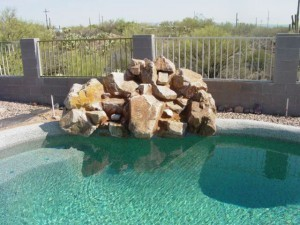# Swimming Pool Water Volume In Gallons

Knowing the volume of water in the pool is necessary forproper maintenance. Without this information, you could end up putting too much or too little cleaning agents.

### Swimming Pool Water Volume in Gallons: 48 Inch Walls Above Ground

The 12 ft round pool has 2,975 gallons while the 15 ft round has 4,646 gallons. The 18 ft round has 7,646 gallons; the 21 ft round has 9,106 gallons and the 24 ft round can carry 11,895 gallons.

The 27 ft round pool has 15,054 gallons and the 30 ft round has 18,585 gallons. The 33 ft round has 22,488 gallons. A 12′ x 24′ oval has 5,948 gallons while the 15′ x 30′ oval has 9,293 gallons. The 16′ x 32′ holds 10,573 gallons and the 18′ x 33′ 12,267 gallons.

### Swimming Pool Water Volume in Gallons: 52 Inch Wall Above Ground

The round pool measurements are as follows: for 12 ft, it is 3,398 gallons; for 15 ft it is 5,310 gallons and for 18 ft it is 8,602 gallons. For 21 ft round pools, the volume is 10,408 gallons and for 24 ft pools it is 13,594 gallons.

For the 27 ft type it is 17,205 gallons; for the 30 ft it is 21,240 gallons and for the 33 ft it is 25,700 gallons. For the oval types: for the 12′ x 24′, it is 6,797 gallons; for the 15′ x 30′ it is 10,620 gallons and for the 16′ x 32′ it is 12,084 gallons. The 18′ x 33′ oval is 14,019 gallons and for the 18′ x 40′ it is 19,116 gallons.

### Swimming Pool Water Volume in Gallons: In-Ground Pool Average Depth (3.5 Gal)

For a pool size measuring 12′ x 24′, the average depth is 7,600. For the 14′ x 28′ pool it is 10,300 gallons while for the 15′ x 30′ it is 11,800. The depth of a 16′ x 32′ pool is 13,400 and for 18′ x 36′ it is 17,000.

The 19′ x 38′ pool holds 19,000 gallons and the 20′ x 40′, 21,000. The 22′ x 44′ pool has a gallon depth of 25,400. The 25′ x 45′ pool has a depth of 29,531 gallons. The 25′ x 50′ in-ground pool has 32,800 gallons and the 30′ x 50 swimming pool 39,375 gallons.

### Swimming Pool Water Volume in Gallons: In-Ground Pool Average Depth (4.5 Gal)

For the 12′ x 24′ it is 9,700 gallons; for the 14′ x 28′ pool it is 13,200; for the 15′ x 30′ it is 15,200. An in-ground pool measuring 16′ x 32′ has a depth of 17,300. An 18′ x 36′ pool has 21,900 gallons deep and the 19′ x 38′ pool 24,400 gallons.

A pool measuring 20′ x 40′ holds 27,000 gallons. The 22′ x 44′ pool is 32,700. A 25′ x 45′ pool has 37,968 gallons. The average depth of a 25′ x 50′ pool is 42,200.

To figure out the swimming pool water volume in gallons yourself, multiply the length, width, average depth and the multiplier. The multiplier is 7.5 for square, free form and rectangular pools. For round and ovals it’s 5.9.